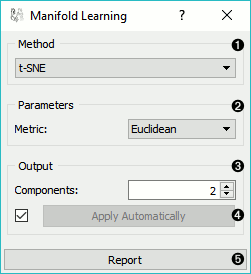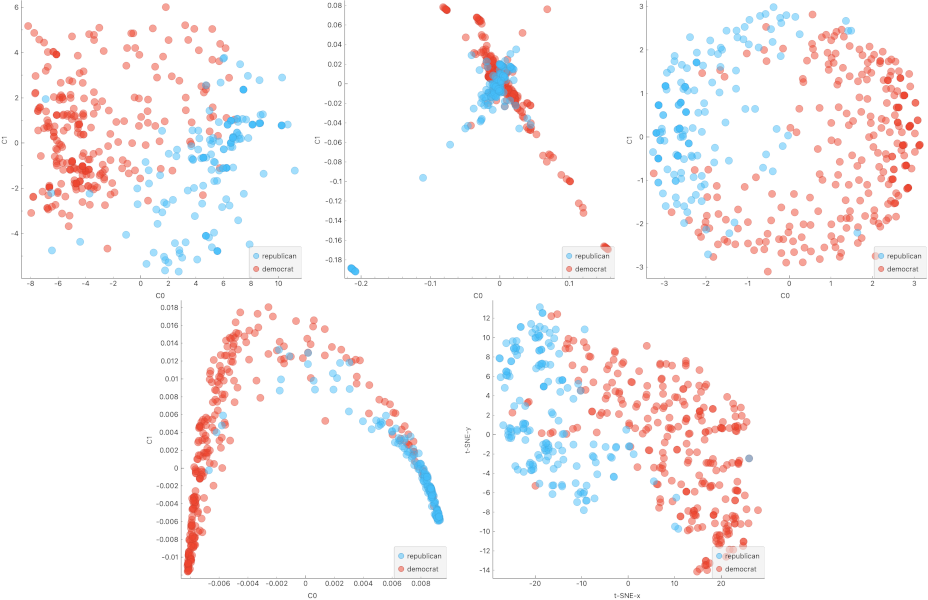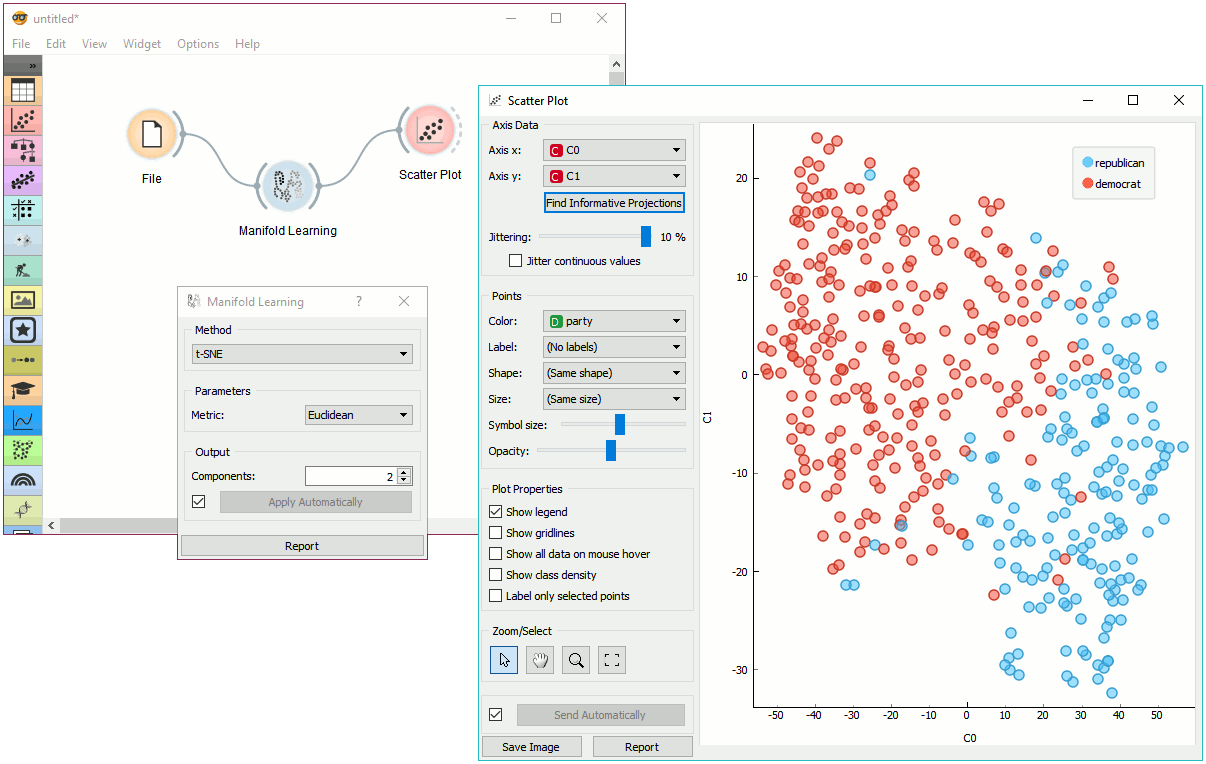# Manifold Learning

Nonlinear dimensionality reduction.

Inputs

• Data: input dataset

Outputs

• Transformed Data: dataset with reduced coordinates

Manifold Learning is a technique which finds a non-linear manifold within the higher-dimensional space. The widget then outputs new coordinates which correspond to a two-dimensional space. Such data can be later visualized with Scatter Plot or other visualization widgets.1. Method for manifold learning:
2. Set parameters for the method:
• t-SNE (distance measures):
• Euclidean distance
• Manhattan
• Chebyshev
• Jaccard
• Mahalanobis
• Cosine
• MDS (iterations and initialization):
• max iterations: maximum number of optimization interactions
• initialization: method for initialization of the algorithm (PCA or random)
• Isomap:
• number of neighbors
• Locally Linear Embedding:
• Spectral Embedding:
• affinity:
• nearest neighbors
• RFB kernel
3. Output: the number of reduced features (components).
4. If Apply automatically is ticked, changes will be propagated automatically. Alternatively, click Apply.
5. Produce a report.

Manifold Learning widget produces different embeddings for high-dimensional data.From left to right, top to bottom: t-SNE, MDS, Isomap, Locally Linear Embedding and Spectral Embedding.

## Preprocessing

All projections use default preprocessing if necessary. It is executed in the following order:

• continuization of categorical variables (with one feature per value)
• imputation of missing values with mean values

To override default preprocessing, preprocess the data beforehand with Preprocess widget.

## Example

Manifold Learning widget transforms high-dimensional data into a lower dimensional approximation. This makes it great for visualizing datasets with many features. We used voting.tab to map 16-dimensional data onto a 2D graph. Then we used Scatter Plot to plot the embeddings.